# 数据结构与算法 - 哈夫曼树## 哈夫曼（David Huffman）PS：貌似人家不是程序员，人家是科学家好吧….

## 哈夫曼编码背景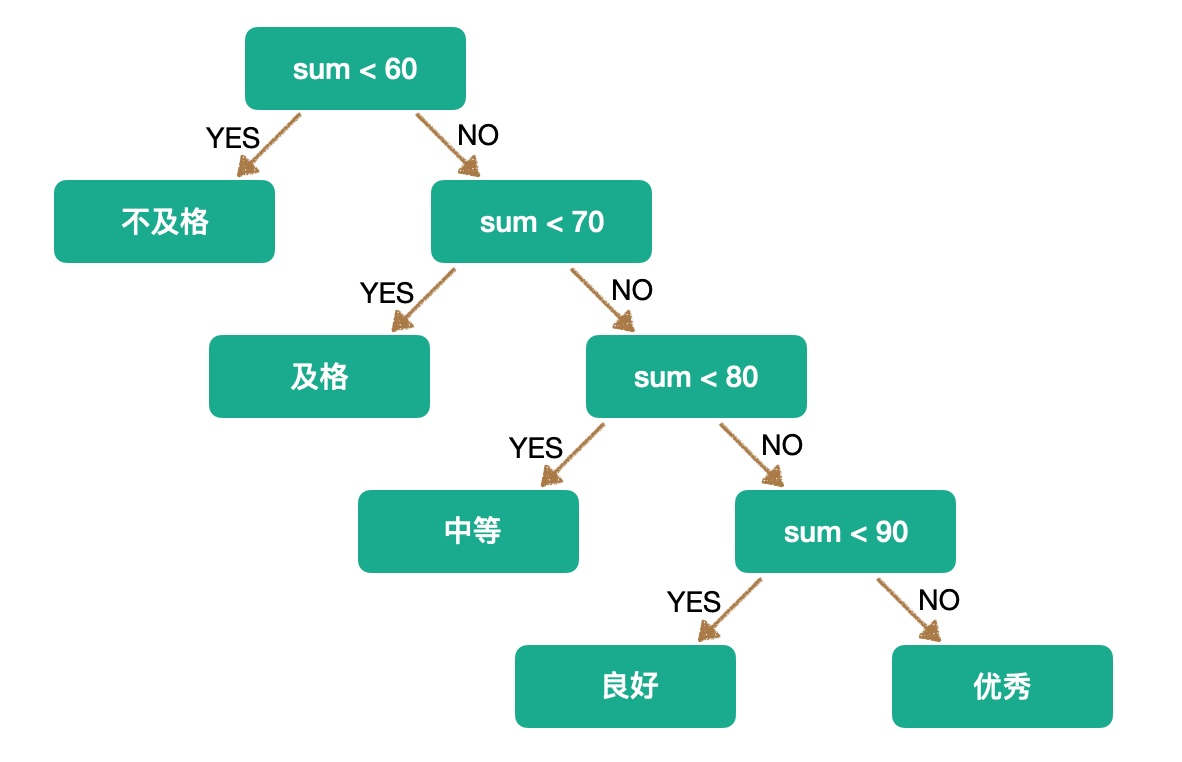## 哈夫曼思考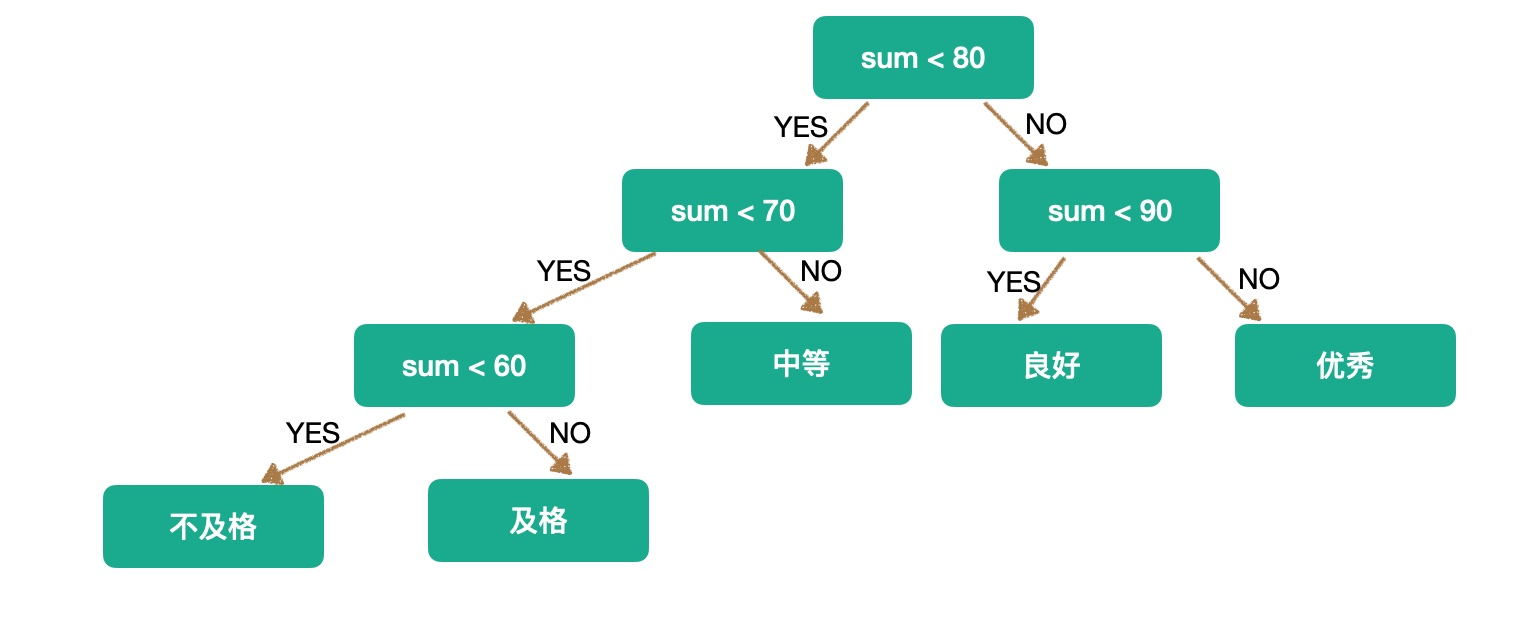## 哈弗曼树的构建

1. 首先找到目前权值最小的两个子几点，构成一个二叉树，如下：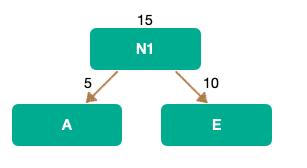1. 由于A和E生成一个二叉树，其根节点的权值就会变成 A+E = 15 ，所以接下来 我们就要从 N1,B,D,C 中找到最小的两个，PS：其原理就是每次都要找最小的两个权值生成树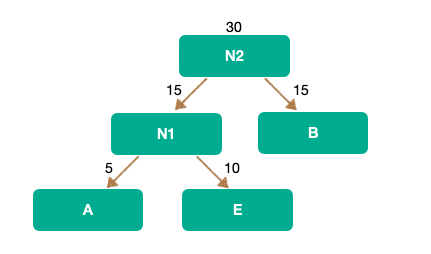1. 以此类推，最终这个哈弗曼树应该是这样：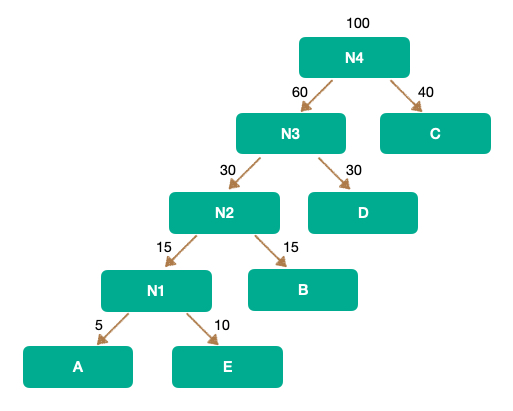## 代码

``const int MaxValue = 10000;//初始设定的权值最大值const int MaxBit = 4;//初始设定的最大编码位数const int MaxN = 10;//初始设定的最大结点个数typedef struct HaffNode{    int weight;    int flag;    int parent;    int leftChild;    int rightChild;}HaffNode;typedef struct Code//存放哈夫曼编码的数据元素结构{    int bit[MaxBit];//数组    int start;  //编码的起始下标    int weight;//字符的权值}Code;//1.//根据权重值,构建哈夫曼树;//{2,4,5,7}//n = 4;void Haffman(int weight[],int n,HaffNode *haffTree){    int j,m1,m2,x1,x2;    //1.哈夫曼树初始化    //n个叶子结点. 2n-1    for(int i = 0; i < 2*n-1;i++){        if(i<n)            haffTree[i].weight = weight[i];        else            haffTree[i].weight = 0;        haffTree[i].parent = 0;        haffTree[i].flag = 0;        haffTree[i].leftChild = -1;        haffTree[i].rightChild = -1;    }    //2.构造哈夫曼树haffTree的n-1个非叶结点    for (int i = 0; i< n - 1; i++){         m1 = m2 = MaxValue;         x1 = x2 = 0;        //2,4,5,7        for (j = 0; j< n + i; j++)//循环找出所有权重中，最小的二个值--morgan        {            if (haffTree[j].weight < m1 && haffTree[j].flag == 0)            {                m2 = m1;                x2 = x1;                m1 = haffTree[j].weight;                x1 = j;            } else if(haffTree[j].weight<m2 && haffTree[j].flag == 0)            {                m2 = haffTree[j].weight;                x2 = j;            }        }        //3.将找出的两棵权值最小的子树合并为一棵子树        haffTree[x1].parent = n + i;        haffTree[x2].parent = n + i;        //将2个结点的flag 标记为1,表示已经加入到哈夫曼树中        haffTree[x1].flag = 1;        haffTree[x2].flag = 1;        //修改n+i结点的权值        haffTree[n + i].weight = haffTree[x1].weight + haffTree[x2].weight;        //修改n+i的左右孩子的值        haffTree[n + i].leftChild = x1;        haffTree[n + i].rightChild = x2;    }}/* 9.2 哈夫曼编码 由n个结点的哈夫曼树haffTree构造哈夫曼编码haffCode //{2,4,5,7} */void HaffmanCode(HaffNode haffTree[], int n, Code haffCode[]){    //1.创建一个结点cd    Code *cd = (Code * )malloc(sizeof(Code));    int child, parent;    //2.求n个叶结点的哈夫曼编码    for (int i = 0; i<n; i++)    {        //从0开始计数        cd->start = 0;        //取得编码对应权值的字符        cd->weight = haffTree[i].weight;        //当叶子结点i 为孩子结点.        child = i;        //找到child 的双亲结点;        parent = haffTree[child].parent;        //由叶结点向上直到根结点        while (parent != 0)        {            if (haffTree[parent].leftChild == child)                cd->bit[cd->start] = 0;//左孩子结点编码0            else                cd->bit[cd->start] = 1;//右孩子结点编码1            //编码自增            cd->start++;            //当前双亲结点成为孩子结点            child = parent;            //找到双亲结点            parent = haffTree[child].parent;        }         int temp = 0;        for (int j = cd->start - 1; j >= 0; j--){            temp = cd->start-j-1;            haffCode[i].bit[temp] = cd->bit[j];        }        //把cd中的数据赋值到haffCode[i]中.        //保存好haffCode 的起始位以及权值;        haffCode[i].start = cd->start;        //保存编码对应的权值        haffCode[i].weight = cd->weight;    }}int main(int argc, const char * argv[]) {    // insert code here...    printf("Hello, 哈夫曼编码!\n");    int i, j, n = 4, m = 0;    //权值    int weight[] = {2,4,5,7};    //初始化哈夫曼树, 哈夫曼编码    HaffNode *myHaffTree = malloc(sizeof(HaffNode)*2*n-1);    Code *myHaffCode = malloc(sizeof(Code)*n);    //当前n > MaxN,表示超界. 无法处理.    if (n>MaxN)    {        printf("定义的n越界，修改MaxN!");        exit(0);    }    //1. 构建哈夫曼树    Haffman(weight, n, myHaffTree);    //2.根据哈夫曼树得到哈夫曼编码    HaffmanCode(myHaffTree, n, myHaffCode);    //3.    for (i = 0; i<n; i++)    {        printf("Weight = %d\n",myHaffCode[i].weight);        for (j = 0; j<myHaffCode[i].start; j++)            printf("%d",myHaffCode[i].bit[j]);        m = m + myHaffCode[i].weight*myHaffCode[i].start;         printf("\n");    }    printf("Huffman's WPS is:%d\n",m);    return 0;}``
------------- 本文结束  感谢您的阅读 -------------